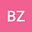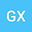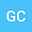The First Integral and Analytical Solution of Chazy Equation with Movable Singular Line
•••Guoliang Xu
Luoyang Normal University
Author ProfileGuangbo Chen
Inner Mongolia University of Science and Technology
Author Profile## Abstract

The basic object of investigation is classical Chazy - a nonlinear third-order autonomous differential equation with movable singular line. In this paper, the first integral of Chazy equation is obtained with the help of Backlund transformation, which is a transcendental dependence on the function and its derivatives . The general solution of equation is represented by Laurent series in the punctured neighborhood of point at infinity, and in the domain is represented by Taylor series. It is proved that the obtained Laurent and Taylor series all converge absolutely in their defined domains. Under the situation that the analytic continuation of solutions of differential equation with movable singular line cannot be realized, a necessary and sufficient condition is presented for obtaining the two-parameter rational solution of Chazy equation from its general solution constructed by Taylor series. And the research results can be applied in the analytic theory of ordinary differential equations.